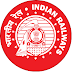## Some of Maths/Quants Questions Asked in RRB NTPC 2016 Exam (All Shifts)

Hello friends,

Many candidates asked us for Maths/Arithmetics & Reasoning Questions asked in RRB NTPC 2016 exam, in this post we are sharing some of the quant questions asked in RRB NTPC CBT exam 2016. These Questions are compiled from feedback of candidates who had appeared in the said exam. This post include all shift questions of 28,29 &30 March 2016 exam. We'll update this page by adding more questions, keep visiting.

Maths and Reasoning Questions asked in the RRB NTPC Exam 2016 (28-30th March)

1. 13 : 30 :: 41:?
Options:
82 ,78,85,80
2.  Sec A+Tan A =1/3,What is Sec A – Tan A ?
3. Train running at 72 kmph how much distance in meters covered in 15 seconds?
4. 100 million = ?
5. A is C,B is D,C is E then what is Z?
6. Tan(-405)= ?
7.  A covers half distance at 3 kmph and other half at doubled speed then how much distance is covered in 5 hrs?
8.  123 : 36 :: 221: ?
9. On a Square 100 tree will plant on every side then how many trees are required?
10.  find the next number 1,1,4,8,9,27,?
11. q=10,fax=50 then Xerox =?
12.  In a certain code ‘PET’ is coded as 64,MET is coded as 52, then SET is coded as 70/73/76/none
13. In total 20 balls 16 balls are of red color,if they are used for  gifts and it should be equal number of balls in each package of gifts, how many gifts are made?
Options (4,5,6,3)
14.  A person sell his radio in 1300 with 30% profit, then what will be the cost price?
15. On selling an article for Rs 270 there is a gain of 12.5 %?What is its cost price?
16. x=2y,x+y=25 what is the value of x?
17.  Series 8,9__,16?
18.  If its 10 am in the clock then minute hand and second hand forms what angle?
19. Price of 107 car is Rs 14,44,500  then what is the price of 1 Car?
20. In a mixture ,ratio of milk and water is 5:3,if amount of milk is 25liters then how much water is there in mixture?
21. Perimeter of rectangle is 48 m and length is double of breadth ,then find the area of rectangle?
22. What is the value of  Sec²Q+2tan*CotQ-tan²Q?
23. cot a=12/5 then (sin a + cos a)cosec a=?
24. find the missing number:  3,4,6,8,12,?18.
25. A man purchases 2 dozen pen at Rs 480 and sell pack of 4 pens of Rs 100 then Find the Profit %?
26. BCA: FGE: ? : NOM
27. How many 5 will occur in 1 to 100?
28. what will be the value of (s+2)³ ?
29. A works double of B and takes 30 days less then B then how many days they both will take to complete the work?
30.  3:9::12:?
31. A covers 40km by 60kmph and 60km by 40kmph,find the average speed?
32. Cost price of a phone is 11000 and selling price is 13500,find the profit %?
33. find the factors of x²-x-132?
34. A can do a work in 10 days& B can do it in 15 days,if they work together for 5 days,then how many days work will be finished?
35. A sum of Rs. 12,500 amounts to Rs. 15,500 in 4 years at the rate of simple interest. What is the rate of interest?
36. if M=14,TANK=61 then STARDOM =?
37. [email protected]=1/√2  then(tan @ + cot @)=?
38. Standard Deviation of set(10,10,10,10,10)?
39.  find the factors of x²+17x+72=0?
40.  Banana at 5 per dozen and orange at  75 per dozen,then find the total price of one and  quarter dozen banana and ¾ dozen orange prize?
41.  Value of tan 1125?
42. What is Range of 11,13,18,28,19,18,12,19 ?

maths questions asked in RRB NTPC CBT 2016Reviewed by Admin on 10:49:00 PM Rating: 5